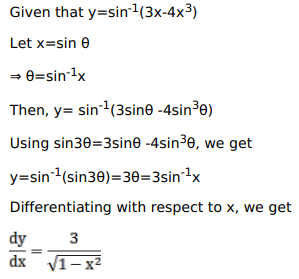# Solve this following

Question:

Mark $(\sqrt{)}$ against the correct answer in the following:

If $y=\sin ^{-1}\left(3 x-4 x^{3}\right)$ then $\frac{d y}{d x}=?$

A. $\frac{3}{\sqrt{1-x^{2}}}$

B. $\frac{-4}{\sqrt{1-x^{2}}}$

C. $\frac{3}{\sqrt{1+\mathrm{x}^{2}}}$

D. none of these

Solution: